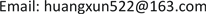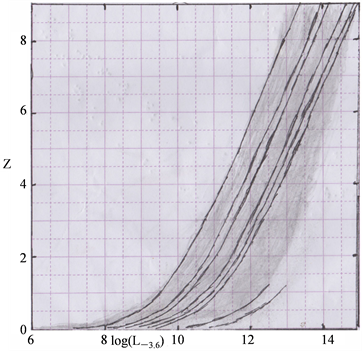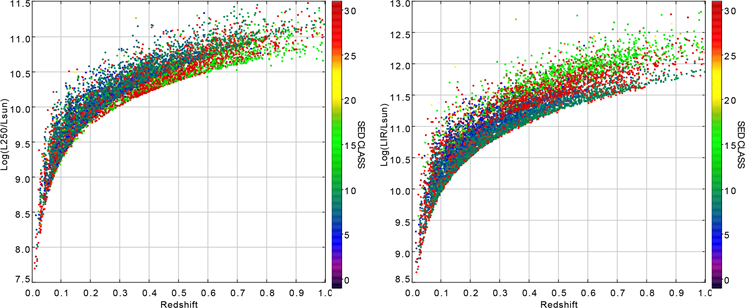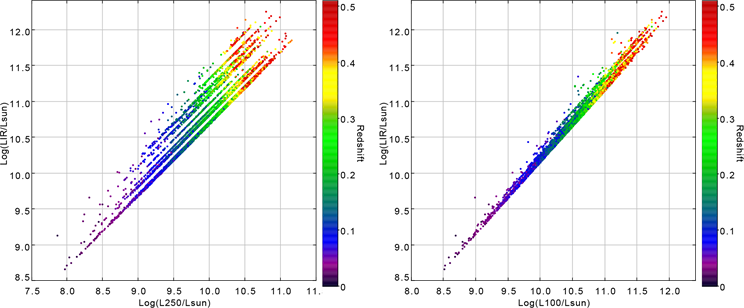﻿ 星系的近似等光度概率和光度图的线性统计分析 Galaxy’s Approximate Luminosity Probability and Linear Statistic Analysis of Luminosity Graph

Astronomy and Astrophysics
Vol.06 No.02(2018), Article ID:24552,18 pages
10.12677/AAS.2018.62003

Galaxy’s Approximate Luminosity Probability and Linear Statistic Analysis of Luminosity Graph

Xun Huang*

Ningjiang Middle School in Xingning, Xingning GuangdongReceived: Mar. 31st, 2018; accepted: Apr. 19th, 2018; published: Apr. 26th, 2018ABSTRACT

Electromagnetic band complies with smooth function, when galaxy’s luminosity is at Z > 0.0041. Approximate luminosity exists in Z-logL graph. Approximate luminosity verifies that when galaxy’s luminosity is fixed value, calculating fixed luminosity has nothing to do with distance (or redshift). When some fixed luminosity is over certain distance that can’t be observed, even university’s extremely weak gravitational effect is one of the conditions that make dark matter exist. Only with logLλ1-logLλ2 graph, we can’t learn the compactness or dispersion of point set on the diagonal line. But it can be learned by linear statistics. Galaxy’s electromagnetic wave has very weak gravitational effect during long-distanced transmission. When the wavelength shortens by exp(Z/2), luminosity must increase by exp(Z/2). Analysis of galaxy’s approximate luminosity and linear relation of logLλ1-logLλ2 is more accurate than the analysis and validation of two data’s above. It replenishes the examples of galaxy group mainly based on galaxy great wall network.

Keywords:Redshift-Luminosity Graph, Linear Statistic of L-L Graph, Approximate Luminosity Sample Statistic Variance, New Gravity Cosmos Metric, Galaxy Great Wall Network1. 前言

2. 基础方程的列出

$\nu S\left(chZ-1\right)={\nu }_{n}{S}_{n}\left(ch{Z}_{n}-1\right)$ .(1)

${S}_{n}=\frac{\nu S\left(chZ-1\right)}{{\nu }_{n}\left(ch{Z}_{n}-1\right)}$ . (2)

${Z}_{n}=arch\left[\frac{\nu S\left(chZ-1\right)}{{\nu }_{n}{S}_{n}}+1\right]$ . (3)

$r=200\left(1-{\text{e}}^{-Z}\right)$ ，单位：亿光年。 (4)

$t=200Z$ ，单位：亿年。 (5)

${L}_{\mu m}=4\text{π}{d}_{L}^{2}\left(c/{\lambda }_{\mu m}\right)\left({10}^{-32}{S}_{\mu m}\right)$ ，其中 ${d}_{L}^{2}=2{r}_{s}^{2}\left(chZ-1\right)$(6)

${L}_{\lambda }=4\text{π}{S}_{\lambda }\left(或{F}_{\lambda }\right)\left(c/\lambda \right){d}_{L}^{2}$${L}_{\nu }=4\text{π}\nu {S}_{\nu }\left(或{F}_{\nu }\right){d}_{L}^{2}$ (6a)

$\mathrm{log}\left({L}_{3.6}/{L}_{sun}\right)=9.29186+\text{log}\left[{S}_{3.6}\left(\mathrm{cosh}Z-1\right)\right]$ . (7)

$\mathrm{log}\left({L}_{4.5}/{L}_{sun}\right)=9.19495+\mathrm{log}\left[{S}_{4.5}\left(\mathrm{cosh}Z-1\right)\right]$ . (8)

$\mathrm{log}\left({L}_{g}/{L}_{sun}\right)=10.73443+0.4\left(22.5-g\right)+\mathrm{log}\left(\mathrm{cosh}Z-1\right)$ .(9)

$\mathrm{log}\left({L}_{r}/{L}_{sun}\right)=10.6182+0.4\left(22.5-r\right)+\mathrm{log}\left(\mathrm{cosh}Z-1\right)$ . (10)

$\Delta k=2.5\mathrm{log}\left[{\text{e}}^{Z}/\left(1+Z\right)\right]$ . (11)

${m}_{k}=m+2.5\mathrm{log}\left[{\text{e}}^{Z}/\left(1+Z\right)\right]$ . (12)

3. 星系表中红外通量密度及红移近似等红外光度计算分析Table 1 . Pick fixed value Full 142 in galaxy tableII/326/zcatrev. When flux density S3.6 = 346.29, Z = 0.762, log(L3.6) = 11.3151 or L3.6 = 2.1662 × 1011Lsun, When S4.5 = 221.61, log(L4.5) = 11.0243.When Magnitude gmag = 21.98, rmag = 20.52. log(Lg) = 10.4262, log(Lr) = 10.8940. When k is revised to gmag = 22.19, rmag = 20.72, log(Lgn) = 10.3422, log(Lrn) = 10.8140. When the galaxy is 10.6654 billion light year away from the earth, it needs 152.4 light years to transmit the luminosity, 22 valuesTable 2 .Basing on the first column in Table II/326/zcatrev, according to Equation (2), to find 24 corresponding values S3.6n and Zn of S3.6 = 24.70 and Z = 0.914Figure 1. Theoretically draw redshift-infrared 3.6 μm luminosity graphTable 3. Luminosity’s average log L ¯ of Table 1, overall variance σ2L, standard variance σLTable 4. Luminosity’s average log L ¯ of Table 2, overall variance σ2L, standard variance σL

$\nu S\left(chZ-1\right){\text{e}}^{Z/2}={\nu }_{n}{S}_{n}\left(ch{Z}_{n}-1\right){\text{e}}^{{Z}_{n}/2}$ ,(13)

${S}_{n}=\frac{\mu S\left(chZ-1\right){\text{e}}^{Z/2}}{{\nu }_{n}\left(ch{Z}_{n}-1\right){\text{e}}^{{Z}_{n}/2}}$ , (14)

${L}_{\mu m}=4\text{π}{d}_{L}^{2}\left[c/\left({\lambda }_{\mu m}{\text{e}}^{-Z/2}\right)\right]\left({10}^{-32}{S}_{\mu m}\right)$ . (15)

$\mathrm{log}\left({L}_{3.6}/{L}_{sun}\right)=9.29186+\mathrm{log}\left[{S}_{3.6}\left(chZ-1\right){\text{e}}^{Z/2}\right]$ . (16)

$\mathrm{log}\left({L}_{4.5}/{L}_{sun}\right)=9.19495+\mathrm{log}\left[{S}_{4.5}\left(chZ-1\right){\text{e}}^{Z/2}\right]$ . (17)

$\mathrm{log}\left({L}_{g}/{L}_{sun}\right)=10.73443+0.4\left(22.5-g\right)+\mathrm{log}\left[\left(chZ-1\right){\text{e}}^{Z/2}\right]$ . (18)

$\mathrm{log}\left({L}_{r}/{L}_{sun}\right)=10.6182+0.4\left(22.5-r\right)+\mathrm{log}\left[\left(chZ-1\right){\text{e}}^{Z/2}\right]$ . (19)Table 5.30 data is based on 142*, the others are sought according to Equation (14). And then to calculate the luminosity of each wave bandTable 6.41 data is based on 42*, the others are sought according to Equation(14). Luminosity increases by exp(Z/2). And then to calculate the luminosity of each wave bandTable 7. Luminosity’s average log L ¯ of Table 5, overall variance σ2L, standard variance σLTable 8. Luminosity’s average log L ¯ of Table 6, overall variance σ2L, standard variance σL

4. 星系红外光度logLλ1-logLλ2线性分析

4.1. 星系红外光度线性分析

$\mathrm{log}\left({L}_{5.8}/{L}_{sun}\right)=9.08473+\mathrm{log}\left[{S}_{5.8}\left(chZ-1\right)\right]$ (20)

$\mathrm{log}\left({L}_{8}/{L}_{sun}\right)=8.94507+\mathrm{log}\left[{S}_{8}\left(chZ-1\right)\right]$ (21)

$\mathrm{log}\left({L}_{24}/{L}_{sun}\right)=8.46795+\mathrm{log}\left[{S}_{24}\left(chZ-1\right)\right]$ (22)

$\mathrm{log}\left({L}_{5.8}/{L}_{sun}\right)=9.08473+\mathrm{log}\left[{S}_{5.8}\left(chZ-1\right){\text{e}}^{Z/2}\right]$ . (23)

$\mathrm{log}\left({L}_{8。0}/{L}_{sun}\right)=8.94507+\mathrm{log}\left[{S}_{8。0}\left(chZ-1\right){\text{e}}^{Z/2}\right]$ . (24)

$\mathrm{log}\left({L}_{24}/{L}_{sun}\right)=8.46795+\mathrm{log}\left[{S}_{24}\left(chZ-1\right){\text{e}}^{Z/2}\right]$ (25)

$\mathrm{log}\left({L}_{IR}\right)=1.37\left(±0.04\right)×\left(\mathrm{log}{{L}^{\prime }}_{CO}\right)-1.74\left(±0.40\right)$ , $\mathrm{log}\left({L}_{IR}\right)=1.13×\left(\mathrm{log}{{L}^{\prime }}_{CO}\right)+0.53$ .Table 9. Linear statistic log L λ 2 = a ⋅ log L λ 1 + b , confidence level r, n ≤ 72Table 10. Linear statistic log ( L λ 2 ) = a ⋅ log ( L λ 1 ) + b , confidence level r, n ≤ 72 . Each infrared luminosity increases by exp(Z/2)

4.2. 星系可见光度与红外光度线性分析及平均光度方差分布近似正态分布Table 11. Table of linear luminosity and average luminosity of same wavelength. log L λ = a ⋅ log L R + b . r is confidence level. n is quantity of sample, which is same with each other in the same columnTable 12. It’s the statistic of variance σ L ¯ of each galaxy’s average luminosity and they approximately appear to be normal distribution

5. 星系长城局域补充

(简写为A)及后发座Coma Cluster。

$\Delta r={r}_{s}\left({\text{e}}^{-{Z}_{1}}-{\text{e}}^{-{Z}_{2}}\right)$ (26)

$D=\frac{{r}_{s}\theta {\text{e}}^{-Z/2}\left(1-{\text{e}}^{-Z}\right)}{206264.8}$ (27)

(2) http://vizier.u-strasbg.fr/viz-bin/VizieR?-source=J/A%2BA/490/923表13中A1413、AM546-324和A85红移间距文献中可能不很准确，视纵向间距小于60 Mpc，文献中作者们对红移间距测量不很重视，没有上述分析内容。表13中的第3列红移间距有些值原文献中以cz = v表出，现统一用红移表出。充分验证了Z > 0.01的星系集群非团，应该是星系长城局域或星系纤维柱为主要特征。Table 13. 15 Abell Cluster and the first, second, third, fifth column is the data of original literature in the bracket. The fourth and sixth column is calculated value of Equation (1) and (6) in Literature  (of Equation (26) and (27)). (P.S: the bracket in the first and sixth column is references)

6. 小结与讨论

Galaxy’s Approximate Luminosity Probability and Linear Statistic Analysis of Luminosity Graph[J]. 天文与天体物理, 2018, 06(02): 29-46. https://doi.org/10.12677/AAS.2018.62003

1. 1. 黄洵. 新引力宇宙度规在星系光度和星系团的验证[J]. 天文与天体物理, 2016, 4(4): 69-80.

2. 2. 黄洵. 新引力宇宙度规计算星系质量和宇宙物质密度的新分析[J]. 天文与天体物理, 2018, 6(1): 11-27. https://doi.org/10.12677/AAS.2018.61002

3. 3. 黄洵. 新引力宇宙度规与观测数据部分验证[EB/OL]. 中国科学院 科学智慧火花. http://idea.cas.cn/viewdoc.action?docid=40475, 2015-10-25.

4. 4. 黄洵. 经典距离与光锥距离的应用分析[EB/OL]. 中国科学院 科学智慧火花. http://idea.cas.cn/viewdoc.action?docid=38375, 2015-08-17.

5. 5. Carilli, C.L. and Walter, F. Cool Gas in High Redshift Galaxies. 65. [1301.0371v2]. http://lanl.arxiv.org/abs/1301.0371v2

6. 6. Marchetti, L., Vaccari, M., Franceschini, A., et al. (2016) The HerMES Sub-Millimetre Local and Low-Redshift Luminosity Functions MNRAS. Monthly Notices of the Royal Astronomical Society, 456, 1999-2023. http://lanl.arxiv.org/abs/1511.06167v1 https://doi.org/10.1093/mnras/stv2717

7. 7. 向守平, 冯珑珑, 编著. 宇宙大尺度结构的形成[M]. 第2版. 中国科学技术出版社, 2011: 225.

8. 8. Gruppioni, C., Pozzi, F., Polletta, M., et al. (2008) The Contribution of AGNs and Star-Forming Galaxies to the Mid-Infrared as Revealed by Their Spectral Energy Distributions. The Astrophysical Journal, 684. http://iopscience.iop.org/article/10.1086/589848/meta;jsessionid=6B1786A51A205A1C0603D26F515E9A15.c3.iopscience.cld.iop.org http://vizier.u-strasbg.fr/viz-bin/VizieR?-source=J/ApJ/684/136

9. 9. Barrena, R., Boschin, W., et al. (2007) Internal Dynamics of the Radio Halo Cluster Abell 773: A Multiwavelength Analysis. Figure 1, Table 3. http://lanl.arxiv.org/abs/astro-ph/0701833v2

10. 10. Sanchez, S.F., Cardiel, N., et al. (2006) Morphologies and Stellar Populations of Galaxies in the Core of Abell 2218. Table 4, Table 5. http://lanl.arxiv.org/abs/astro-ph/0611660v2

11. 11. Verheijen, M., van Gorkom J.H., et al. (2007) WSRT Ultra-Deep Neutral Hydrogen Imaging of Galaxy Clusters at z = 0.2, a Pilot Survey of Abell 963 and Abell 2192. 2. http://arxiv.org/abs/0708.3853v1

12. 12. Cybulski, R., Yun, M.S., et al. (2015) Early Science with the Large Millimeter Telescope: COOL BUDHIES I—A Pilot Study of Molecular and Atomic Gas at z~0.2. 3, Table 1. http://arxiv.org/abs/1510.08450v1

13. 13. Oh, S., Yi, S.K., et al. The SAMI Galaxy Survey: Galaxy Interactions and Kinematic Anomalies in Abell 119. [1609.03595v1]. http://lanl.arxiv.org/abs/1609.03595v1

14. 14. Petrushevska, T., Amanullah, R., et al. High-Redshift Supernova Rates Measured with the Gravitational Telescope A1689. [1607.01617v3]. http://lanl.arxiv.org/abs/1607.01617v3

15. 15. Barrena, R., Girardi, M. and Boschin, W. (2013) The Puzzling Merging Cluster Abell 1914: New Insights from the Kinematics of Member Galaxies. Table 1, Figure 1. http://arxiv.org/abs/1301.5200v1

16. 16. Faún-dez-Abans, M., Krabbe, A.C., et al. (2012) A Study of the Remarkable Galaxy System AM 546-324 (the Core of Abell S0546). http://lanl.arxiv.org/abs/1206.0719v1

17. 17. Venturi, T., Rossetti, M., et al. (2017) The Two-Component Giant Radio Halo in the Galaxy Cluster Abell 2142. A &A, 603, A125. https://doi.org/10.1051/0004-6361/201630014

18. 18. Tanaka, N., Furuzawa, A., et al. (2010) Suzaku Observations of the Merging Cluster Abell 85: Temperature Map and Impact Direction. Publications of the Astronomical Society of Japan, 62, 743-754. http://lanl.arxiv.org/abs/1006.4328v1 https://doi.org/10.1093/pasj/62.3.743

19. 19. Shim, H., Im, M., et al. (2010) Merging Galaxy Cluster Abell 2255 in Mid-Infrared. http://lanl.arxiv.org/abs/1011.6408v1

20. 20. Cybulski, R., Yun, M.S., et al. (2015) Early Science with the Large Millimeter Tele-scope: COOL BUDHIES I—A Pilot Study of Molecular and Atomic Gas at z~0.2. http://arxiv.org/abs/1510.08450v1

21. 21. Dale, D.A. and Uson, J.M. (2003) Signatures of Galaxy-Cluster Interactions: Tully-Fisher Observations at z~0.1. The Astronomical Journal, 126, 675. http://lanl.arxiv.org/abs/astro-ph/0304371v1 https://doi.org/10.1086/376478

22. 22. Johnston-Hollitt, M., Hunstead, R.W. and Corbett, E. (2007) The Optical Mor-phology of A3667 Re-Examined. http://lanl.arxiv.org/abs/0711.4129v1Figure A1. The left is graph of flux density S250μm infrared luminosity-redshift, the right is graph of common infrared luminosity-redshift. Only graph of Z < 1 can be drawn according to the two diagrams and infrared luminosity diagram of all redshifts can’t be drawn, which is one of the most serious problems in standard cosmology. The two graphs can be extended to 10 times of redshiftFigure A2. The left is graph of $\left({L}_{IR}-{L}_{250\mu \text{m}}\right)$ and the right is graph of $\left({L}_{IR}-{L}_{100\mu \text{m}}\right)$ . Only graph of Z < 0.5 can be drawn according to the two graphs and Graph $\left(L-L\right)$ of all redshifts can’t be drawn. Point coordinates should gather closely on both sides of the diagonal. Especially in the left graph, point coordinates gather far away from the diagonal, which is one of the most serious problems in standard cosmology as well. The two graphs can be extended to 10 times of redshift. L-L graph’s compactness or dispersion can be analyzed by linear statistic

NOTES

*退休。